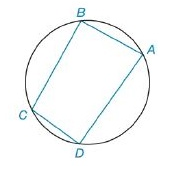Chapter 8.2, Problem 12EElementary Geometry For College St...

7th Edition
Alexander + 2 others
ISBN: 9781337614085

Solutions

Chapter
SectionElementary Geometry For College St...

7th Edition
Alexander + 2 others
ISBN: 9781337614085
Textbook Problem

For Exercises 11 and 12, use Brahmagupta’s Formula.For cyclic quadrilateral A B C D , find the area if A B   =   6 cm, B C   =   7 cm, C D   =   2 cm, and D A   =   9 cm.To determine

To Find:

The area of a cyclic quadrilateral using Brahmagupta’s Formula.

Explanation

Formula Used:

For a cyclic quadrilateral with sides of lengths a,b,c and d, the area A is given by

A=(s-a)(s-b)(s-c)(s-d),

where s=12(a+b+c+d).

Calculation:

Consider, AB=a=6 cm,BC=b=7 cm CD=c=2 cm andAD=d=9 cm.

s=126+7+2+9=12

Still sussing out bartleby?

Check out a sample textbook solution.

See a sample solution

The Solution to Your Study Problems

Bartleby provides explanations to thousands of textbook problems written by our experts, many with advanced degrees!

Get Started

Find more solutions based on key concepts